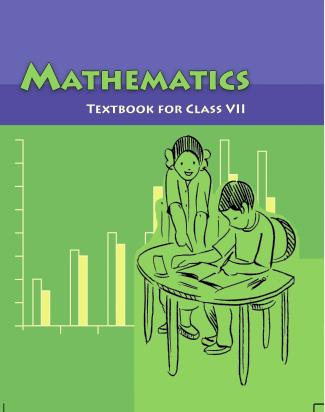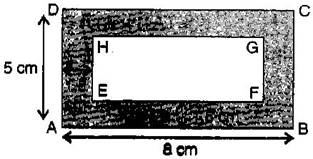1. /
2. CBSE
3. /
4. Class 07
5. /
6. Mathematics
7. /
8. NCERT Solutions for Class...

# NCERT Solutions for Class 7 Maths Exercise 11.1### myCBSEguide App

Download the app to get CBSE Sample Papers 2023-24, NCERT Solutions (Revised), Most Important Questions, Previous Year Question Bank, Mock Tests, and Detailed Notes.

NCERT solutions for Maths Perimeter and Area## NCERT Solutions for Class 7 Maths Perimeter and Area

###### Question 1.The length and breadth of a rectangular piece of land are 500 m and 300 m respectively. Find:

(i) Its area.

(ii) The cost of the land, if 1 {tex}{m^2}{/tex}of the land costs Rs. 10,000.

Given: Length of a rectangular piece of land = 500 m and

Breadth of a rectangular piece of land = 300 m

(i) Area of a rectangular piece of land = Length x Breadth

= 500 x 300 = 1,50,000 {tex}{m^2}{/tex}

(ii) Since, the cost of 1 {tex}{m^2}{/tex}land = Rs. 10,000

Therefore, the cost of 1,50,000 m2 land = 10,000 x 1,50,000

= Rs. 1,50,00,00,000

NCERT Solutions for Class 7 Maths Exercise 11.1

###### Question 2.Find the area of a square park whose perimeter is 320 m.

Given: Perimeter of square park = 320 m

{tex} \Rightarrow {/tex} 4 x side = 320

{tex} \Rightarrow {/tex} side = {tex}\frac{{320}}{4}{/tex} = 80 m

Now, Area of square park = side x side

= 80 x 80 = 6400 {tex}{m^2}{/tex}

Thus, the area of square park is 6400{tex}{m^2}{/tex}.

NCERT Solutions for Class 7 Maths Exercise 11.1

###### Question 3.Find the breadth of a rectangular plot of land, if its area is 440 {tex}{m^2}{/tex}and the length is 22 m. Also find its perimeter.

Area of rectangular park = 440 {tex}{m^2}{/tex}

{tex} \Rightarrow {/tex} length x breadth = 440 {tex}{m^2}{/tex}

{tex} \Rightarrow {/tex} 22 x breadth = 440 {tex} \Rightarrow {/tex} breadth = {tex}\frac{{440}}{{22}}{/tex} = 20 m

Now, Perimeter of rectangular park = 2 (length + breadth)

= 2 (22 + 20)

= 2 x 42 = 84 m

Thus, the perimeter of rectangular park is 84 m.

NCERT Solutions for Class 7 Maths Exercise 11.1

###### Question 4.The perimeter of a rectangular sheet is 100 cm. If the length is 35 cm, find its breadth. Also find the area.

Perimeter of the rectangular sheet = 100 cm

{tex} \Rightarrow {/tex} 2 (length + breadth) = 100 cm

{tex} \Rightarrow {/tex} 2 (35 + breadth) = 100 {tex} \Rightarrow {/tex} 35 + breadth = {tex}\frac{{100}}{2}{/tex}

{tex} \Rightarrow {/tex} 35 + breadth = 50 {tex} \Rightarrow {/tex} breadth = 50 – 35

{tex} \Rightarrow {/tex} breadth = 15 cm

Now, Area of rectangular sheet = length x breadth

= 35 x 15 = 525{tex}c{m^2}{/tex}

Thus, breadth and area of rectangular sheet are 15 cm and 525 {tex}c{m^2}{/tex}respectively.

NCERT Solutions for Class 7 Maths Exercise 11.1

###### Question 5.The area of a square park is the same as of a rectangular park. If the side of the square park is 60 m and the length of the rectangular park is 90 cm, find the breadth of the rectangular park.

Given: The side of the square park = 60 m

The length of the rectangular park = 90 m

According to the question,

Area of square park = Area of rectangular park

{tex} \Rightarrow {/tex} side x side = length x breadth

{tex} \Rightarrow {/tex} 60 x 60 = 90 x breadth

{tex} \Rightarrow {/tex} breadth = {tex}\frac{{60 \times 60}}{{90}}{/tex} = 40 m

Thus, the breadth of the rectangular park is 40 m.

NCERT Solutions for Class 7 Maths Exercise 11.1

###### Question 6. A wire is in the shape of a rectangle. Its length is 40 cm and breadth is 22 cm. If the same wire is rebent in the shape of a square, what will be the measure of each side. Also find which shape encloses more area?

According to the question,

Perimeter of square = Perimeter of rectangle

{tex} \Rightarrow {/tex} 4 x side = 2 (length + breadth)

{tex} \Rightarrow {/tex} 4 x side = 2 (40 + 22) {tex} \Rightarrow {/tex} 4 x side = 2 x 62

{tex} \Rightarrow {/tex} side = {tex}\frac{{2 \times 62}}{4}{/tex} = 31 cm

Thus, the side of the square is 31 cm.

Now, Area of rectangle = length x breadth = 40 x 22 = 880 {tex}c{m^2}{/tex}

And Area of square = side x side = 31 x 31 = 961 {tex}c{m^2}{/tex}

Therefore, on comparing, the area of square is greater than that of rectangle.

NCERT Solutions for Class 7 Maths Exercise 11.1

###### Question 7. The perimeter of a rectangle is 130 cm. If the breadth of the rectangle is 30 cm, find its length. Also, find the area of the rectangle.

Perimeter of rectangle = 130 cm

{tex} \Rightarrow {/tex} 2 (length + breadth) = 130 cm

{tex} \Rightarrow {/tex} 2 (length + 30) = 130 {tex} \Rightarrow {/tex} length + 30 = {tex}\frac{{130}}{2}{/tex}

{tex} \Rightarrow {/tex} length + 30 = 65 {tex} \Rightarrow {/tex} length = 65 – 30 = 35 cm

Now area of rectangle = length x breadth = 35 x 30 = 1050 {tex}c{m^2}{/tex}

Thus, the area of rectangle is 1050 {tex}c{m^2}{/tex}.

NCERT Solutions for Class 7 Maths Exercise 11.1

###### Question 8.A door of length 2 m and breadth 1 m is fitted in a wall. The length of the wall is 4.5 m and the breadth is 3.6 m. Find the cost of white washing the wall, if the rate of white washing the wall is Rs. 20 per{tex}{m^2}{/tex}.Area of rectangular door = length x breadth = 2 m x 1 m = 2 {tex}{m^2}{/tex}

Area of wall including door = length x breadth = 4.5 m x 3.6 m = 16.2 {tex}{m^2}{/tex}

Now, Area of wall excluding door

= Area of wall including door – Area of door

= 16.2 – 2 = 14.2{tex}{m^2}{/tex}

Since, The rate of white washing of 1 {tex}{m^2}{/tex}the wall = Rs. 20

Therefore, the rate of white washing of 14.2{tex}{m^2}{/tex} the wall = 20 x 14.2

= Rs. 284

Thus, the cost of white washing the wall excluding the door is Rs. 284.

## NCERT Solutions for Class 7 Maths Exercise 11.1

NCERT Solutions Class 7 Maths PDF (Download) Free from myCBSEguide app and myCBSEguide website. Ncert solution class 7 Maths includes text book solutions from Class 7 Maths Book . NCERT Solutions for CBSE Class 7 Maths have total 15 chapters. 7 Maths NCERT Solutions in PDF for free Download on our website. Ncert Maths class 7 solutions PDF and Maths ncert class 7 PDF solutions with latest modifications and as per the latest CBSE syllabus are only available in myCBSEguide.

## CBSE app for Students

To download NCERT Solutions for Class 7 Maths, Social Science Computer Science, Home Science, Hindi English, Maths Science do check myCBSEguide app or website. myCBSEguide provides sample papers with solution, test papers for chapter-wise practice, NCERT solutions, NCERT Exemplar solutions, quick revision notes for ready reference, CBSE guess papers and CBSE important question papers. Sample Paper all are made available through the best app for CBSE students and myCBSEguide website.Test Generator

Create question paper PDF and online tests with your own name & logo in minutes.myCBSEguide

Question Bank, Mock Tests, Exam Papers, NCERT Solutions, Sample Papers, Notes

### 11 thoughts on “NCERT Solutions for Class 7 Maths Exercise 11.1”

1. Mujhe kuch bhi samajh nahi aaya

2. Mujhe kuch bhi a samajh nahi aata

3. Its Jst amazing its too easy to study thank u so much for making concept so easy

4. Very good

5. Nice?

6. It is so easy to learn

7. It is so easy to learn it

8. Good site

9. Very good